ICSE Class 9 Maths Sample Question Paper 3 with Answers

Section – A
(Attempt all questions from this Section)

Question 1.
(a) Factorize : 8(a – 2b)2 – 2a + 4b – 1
8 (a – 2b)2 -2a + 4b – 1 = 8 (a- 2b)2 -2(a – 2b) – 1
a – 2b = x
Then, the expression becomes
= 8x2 – 2x – 1
= 8x2 – 4x + 2x – 1
= 4x (2x – 1) + 1 (2x – 1)
= (2x – 1) (4x + 1)
= {2 (a- 2b) – 1} {4 (a – 2b) + 1} (Putting x-a-2b)
= (2a – 4b – 1) (4a- 8b + 1)(b) The mean of 6 observations is 17.5. If five of them are 14, 9, 23, 25 and 10, find the sixth observation.
Mean of 6 observations is 17.5
5 observations are 14, 9, 23, 25, 10
Let 6th observation be x(c) If θ is an acute angle and sin θ = cos θ, find the value of 2 tan2 θ + sin2 θ-1.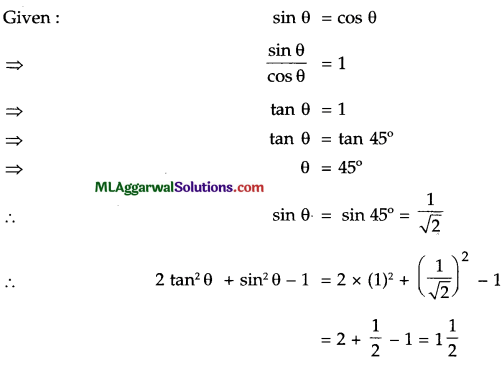Question 2.
(a) A man borrowed ₹15,000 for 2 years. The rate of interest for the two successive years are 8% and 10% respectively. If he repays ₹6,200 at the end of first year, find the outstanding amount at the end of the second year.
(a) For 1st year : P = ₹ 15,000, R = 8% p.a.
$$\mathrm{I}=\frac{15000 \times 8 \times 1}{100}$$ = ₹ 16,200
A = P + I = 15,000 + 1,200 =₹16,200
Amount of money repaid = ₹ 6,200
For 2nd year : P = 16,200 – 6,200 = ₹10,000, R = 10% p.a.
$$\mathrm{I}=\frac{10,000 \times 10 \times 1}{100}$$ = = ₹1,000
∴ A =P+I=10,000+1,000=11,000
∴ The amount outstanding at the end of 2nd year = 11, 000.

(b) Solve for x if log2 (x2 – 4) = 5.
Given: log2(x2 – 4) = 5
x2 – 4 =25
x2 =32 + 4
X = ±√36= ±6.(c) In the following figure, AB is a diameter of a circle with centre O. If chord AC = chord AD,
prove that (i) arc BC = arc DB (ii) AB is bisector of ∠CAD.(i) Given: chord AC = chord AD
⇒ arc AC = arc AD …(i)
Also, arc ACB = arc ADB (AB is a diameter) …(ii)
Subtracting (i) from (ii),
⇒ arc BC = arc DB. Hence Proved.

(ii) ∵ arc BC = arc BD (Proved above)
∴ ∠BAC = ∠DAB
⇒ AB is bisector of ∠CAD.
Hence Proved.Question 3.
(a) Prove that: $$\frac{2^{n}+2^{n-1}}{2^{n+1}-2^{n}}=\frac{3}{2}$$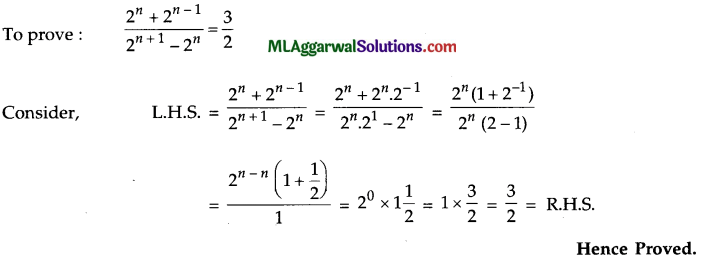(b) Given that 16 cot A = 12, find the value of $$\frac{\sin A+\cos A}{\sin A-\cos A}$$(c) If the altitudes from two vertices of a triangle to the opposite sides are equal, prove that the triangle is isosceles.Question 4.
(a) If a + b + 2c = 0, prove that a3 + b3 + 8c3 – 6abc = 0.
Given : a + b + 2c = 0
⇒ a + b = – 2c
Cubing both sides, we get
(a + b)3 = (- 2c)3
⇒ a3 + b3 + 3ab (a + b) = – 8c3
⇒ a3 + b3 + 3ab (- 2c) = – 8c3
⇒ a3 + b3 + 8c3 – 6abc = 0.
Hence Solved.

(b) Express $$0.1 \overline{34}$$ in the form $$\frac{p}{q}$$ ,p, q ∈ Z and q ≠ 0.
Let x = $$0.1 \overline{34}$$ = 0.1343434…
Multiplying both sides of (i) by 10, we get
10x = 1.343434 ………(i)
Multiplying both sides of (ii) by 100, we get
1000x = 134.3434 ………….(ii)
Subtracting (ii) from (iii), we get
1000x – 10x= 134.3434 … – 1.3434 ……………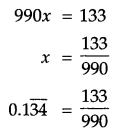(c) If the sides are in the ratio 5 : 3 : 4, prove that it is a right angled triangle.
Ratio of sides = 5:3:4
Let the length of sides be 5x, 3x, 4x.
Here,(3x)2 + (4x)2 = 9x2 + 16x2 = 25x2 = (5x)2
∴ By Pythagoras theorem, the triangle is right angled
Hence Proved.

Section – B
(Attempt any four questions from this Section)

Question 5.
(a) On what sum of money will the difference between compound interest and simple inter­est for 2 years be equal to ₹25 if the rate of interest charged for both is 5% p.a. ?(b) Show by distance formula that the points A (-1, -1), B (2, 3) and C (8,11) are collinear.
Given points are A (-1, -1), B (2, 3), C (8, 11).
Now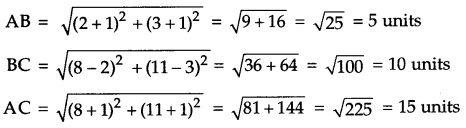AB + BC = 5 + 10 = 15
⇒ AB + BC = AC
The points are collinear.
Hence Proved.(c) Factorize : a6 – 26a3 – 27.
a6 – 26a3 – 27 = a6 – (27 – 1) a3-27 = a6 – 27a3 + a3 – 27
= a3 (a3 – 27) + 1 (a3 – 27) = {a3 – 27) (a3 + 1)
= (a3 – 33) (a3 + 13)
= (a – 3) (a2 + 3a + 9) (a + 1) (a2 – a + 1)

Question 6.
(a) Simplify: $$\frac{\left(x^{a+b}\right)^{2} \cdot\left(x^{b+c}\right)^{2} \cdot\left(x^{c+a}\right)^{2}}{\left(x^{a} \cdot x^{b} \cdot x^{c}\right)^{4}}$$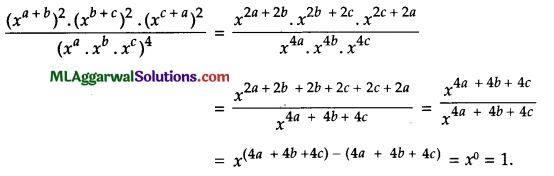(b) In the given figure AABC, D is the mid-point of AB, E is the mid-point of AC. Calculate :
(i) DE, if BC = 8 cm.
(ii) ∠ADE, if ∠DBC = 125°.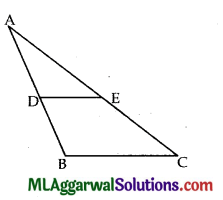Given : D is mid-point of AB, E is the mid-point of AC, BC = 8 cm, ∠DBC = 125°.
The line joining the mid-points of any two sides of a triangle is parallel to the third and is equal the half of it.(c) If a and b are rational numbers, find the values of a and b :
$$\frac{5+2 \sqrt{3}}{7+4 \sqrt{3}}=a+b \sqrt{3}$$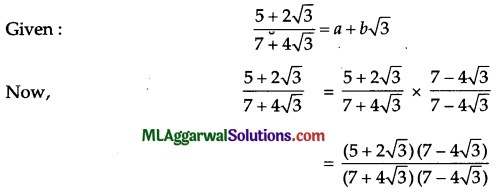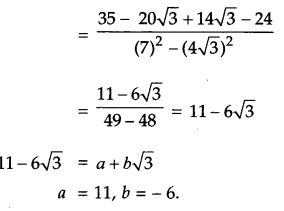Question 7.
(a) Draw a histogram from the following data :

 Weight (in kg) 40-44 45-49 50-54 55-59 60-64 65-69 No. of students 2 8 12 10 6 4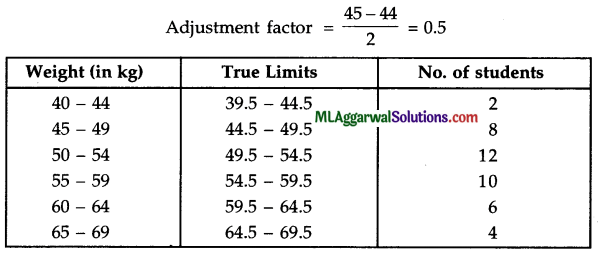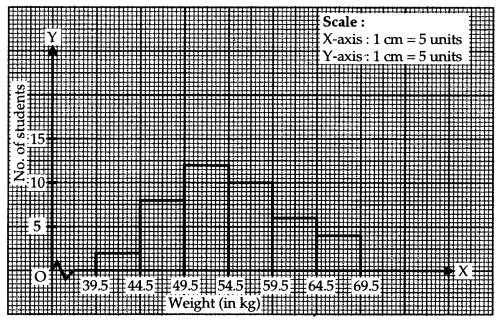(b) Solve:
83x – 67y = 383
67x – 83y = 367.
83x – 67y = 383 ………….(i)
67a – 83y = 367 ………….(ii)
Adding (i) and (ii), we get
150x – 150y = 750
x – y = 5 ………. (iii)
Subtracting (ii) from (i), we get
16x + 16y = 16
x + y = 1 ……… (iv)
Adding (iii) and (iv), we get
2x = 6
X = 3
Putting x = 3 in (iv), we get
3 + y = 1
⇒ y = 1 – 3 = -2.
x = 3, y = – 2(c) In the following figure, area of parallelogram AFEC is 140 cm2. State, giving reason, the area of (i) parallelogram BFED (ii) ABFD.Given : Area of parallelogram AFEC = 140 cm2.
(i)  Area of parallelogram BFED = Area of parallelogram AFEC
( ∵ They are on same base and between same parallels)
= 140 cm2

(ii) Area of Δ BFD = $$\frac{1}{2}$$ x Area of parallelogram BFED
(∵ They are on same base and between same parallels)
$$\frac{1}{2}$$ x 140 cm2 = 70 cm2.

Question 8.
(a) If log10 a = m and log10 b = n, express $$\frac{a^{3}}{b^{2}}$$ in terms of m and n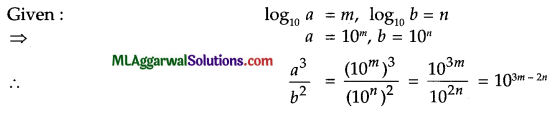(b) Draw the graph of 2x + y = 6 and 2x – y + 2 = 0. Hence, find the area of the region bounded by these lines and X-axis.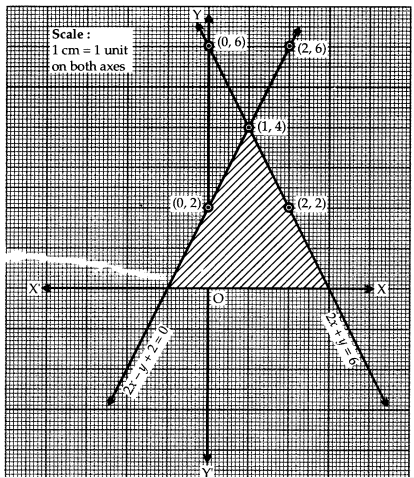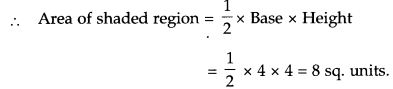(c) Factorize : $$8 x^{3}-\frac{1}{27 y^{3}}$$
Answre: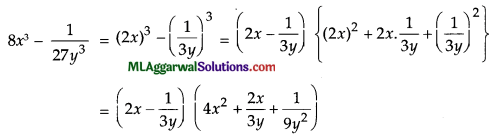Question 9.
(a) If $$\frac{x^{2}+1}{x}=2 \frac{1}{2}$$ find the values of $$(i) x-\frac{1}{x} (ii) x^{3}-\frac{1}{x^{3}}$$(b) In the following figure, OAB is a quadrant of a circle. The radius OA = 3.5 cm and OD = 2 cm. Calculate the area of the shaded portion.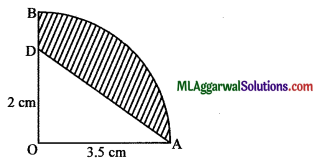Given : OA = 3.5 cm, OD = 2 cm
Area of shaded region = Area of quadrant AOB – Area of ΔAOD.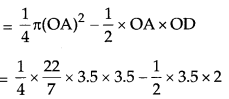= 9.625 – 3.5 = 6.125 cm2.

(c) If a + b + c = 9 and ab + be + ca = 40, find the value of a2 + b2 + c2.
Given: a + b + c = 9 ab + bc + ca = 40
We know, (a+b+c)2 =a2+ b2 + c2 +2(ab+bc+ca)
(9)2 =a2+ b2 + c2+ 2 x 40
a2+ b2 + c2 = 81 – 80 = 1Question 10.
(a) Solve: $$(\sqrt{2})^{2 x+4}=8^{x-6}$$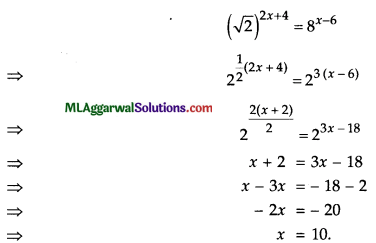(b) Construct a rhombus whose diagonals are 5 cm and 6.8 cm.
Given, diagonals are 5 cm and 6.8 cm.
Steps of construction :
(1) Draw AC = 5 cm.
(2) Draw perpendicular bisector PQ of AC which intersect AC at O.
(3) From POQ, cut-off OB = OD = $$\frac{6.8}{2}$$ = 3.4 cm.
(4) Join the points A, B, C, D.
Then, ABCD is the required rhombus.(c) In a quadrilateral ABCD, AO and BO are the bisectors of ∠A and ∠B respectively.
Prove that ∠AOB = $$\frac{1}{2}$$ (∠C + ∠D).
Given, AO and BO are bisectors of ∠A and ∠B respectively.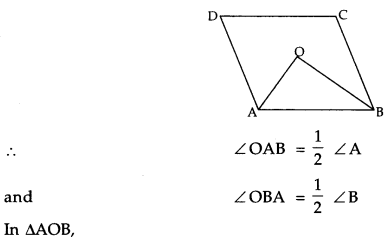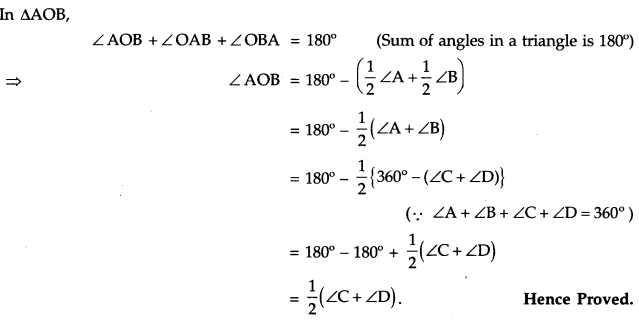Question 11.
(a) Find the value of log5√5 (125).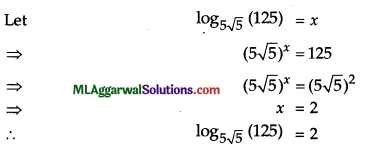(b) The sum of a two-digit number and the number obtained by reversing the order of its digits is 165. If the digits differ by 3, find the number.
Let the digits in tens and units place be x and y respectively.
The number = 10x + y
The number obtained by reversing digits = 10y + x By 1st condition,
(10 + y) + (10y + x) = 165
⇒ 11x + 11y = 165
⇒ x + y =15 …(ii)
By 2nd condition, x-y =3 …(iii)
or y-x =3 …(iv)
Adding (ii) and (iii), we get 2x = 18
⇒ x =9
Putting x = 9 in (ii), we get y = 15 – 9 = 6
Again, adding (ii) and (iv), we get
2 y =18
⇒ y =9
Putting y = 9 in (ii), we get x = 15 – 9 = 6.
Substituting these values in (i), we get
The number = 10 x 9 + 6 or 10 x 6 + 9 = 96 or 69(c) If the area of an equilateral triangle is 81√3 cm2, find its perimeter.
Let the length side of each of equilateral triangle be a cm.